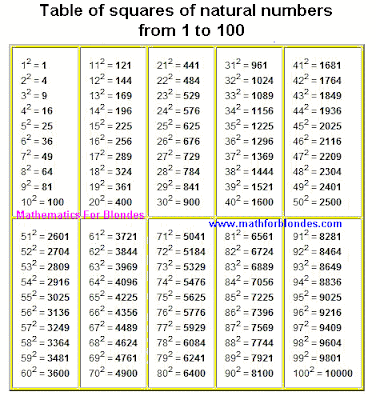## 11.08.2011

### Table of squares of natural numbersIn the table of squares of natural numbers the squares of numbers are presented from 1 to 100. Index of exponent of number as a number two writtenin on the right in the top of that number that is involuted. If a number is erected in a square, it means that this number needs to be increased on a such is exact number.

For example, ten in a square equals one hundred, because if ten to multiplication on ten, for us will turn out one hundred. Five in the second degree equals twenty five, because all know that five at five "again twenty five". And two in a square it not that another, "as twice two four".

Why was such thought of is a exponent of number? For short of record of mathematical expressions, whatever to repeat several times multiplication of identical numbers. If at erection of number in the second exponent we save a quite small place, then at erection of numbers in great exponents the economy of place turns out rather significant.

By the feature of squares of numbers, as well as all other even degrees of number, there is that after involution negative number a positive number ensues. In fact minus on minus gives a plus at an multiplication, how many times we this focus did not repeat. A negative number is in the second, fourth, sixth, eighth et cetera degrees gives a positive number as a result.